# Gauge Transformations: Can G(t,q) be Expressed Differently?

• dynamicskillingme
Since these two expressions are equal, you can interchange the order of the derivatives. In summary, when working with functions of q and t, the order of derivatives can be interchanged as long as the function only depends on q and t. This can be seen through the use of the chain rule and the fact that the two expressions for \frac{\partial \dot{G}}{\partial q} and \frac{d}{dt} \frac{\partial G}{\partial q} are equal.

## Homework Statement

For a gauge function G(t,q) where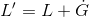,
does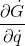or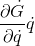have any alternative form or can they be expressed in any other way?

## The Attempt at a Solution

Could you give a little more context to your question? I assume you're talking about electromagnetism? Or are you talking about quantum field theory? What is $L$ and $L'$?

Its a mechanical system, but just working on proofs and theory so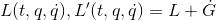I'm working on proving how different quantities change, such as the euler lagrange equations, the generalised momenta, mechanical energy and power

So for example for the euler lagrange I have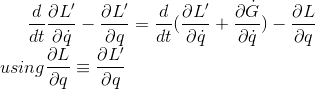correction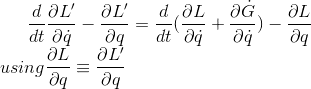Oh, so you're just talking about Lagrangian mechanics.

If $G$ is a function of $q$ and $t$, then you have:

$\dot{G} = \frac{\partial G}{\partial q} \dot{q} + \frac{\partial G}{\partial t}$

So in that case, $\frac{\partial \dot{G}}{\partial \dot{q}} = \frac{\partial G}{\partial q}$

Oh wow, this is painful how much I overlooked that. Thanks!

stevendaryl said:
Oh, so you're just talking about Lagrangian mechanics.

If $G$ is a function of $q$ and $t$, then you have:

$\dot{G} = \frac{\partial G}{\partial q} \dot{q} + \frac{\partial G}{\partial t}$

So in that case, $\frac{\partial \dot{G}}{\partial \dot{q}} = \frac{\partial G}{\partial q}$

A quick question about the same function, would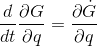be a true statement?

dynamicskillingme said:
A quick question about the same function, wouldbe a true statement?

Yes, if $G$ is only a function of $q$ and $t$.

stevendaryl said:
Yes, if $G$ is only a function of $q$ and $t$.

G is a function of q and t, (G(t,q) to be exact). Could you explain why you can change the order of the derivatives in this case?

dynamicskillingme said:
G is a function of q and t, (G(t,q) to be exact). Could you explain why you can change the order of the derivatives in this case?

If you have a function $X(t,q)$of $q$ and $t$, then $\dot{X} = \frac{d}{dt} X = (\frac{\partial}{\partial t} + \frac{\partial}{\partial q} \frac{dq}{dt}) X = (\frac{\partial}{\partial t} + \frac{\partial}{\partial q} \dot{q}) X$

So $\dot{G} = \frac{\partial G}{\partial t} + \dot{q} \frac{\partial G}{\partial q}$. Therefore, $\frac{\partial \dot{G}}{\partial q} = \frac{\partial^2 G}{\partial t \partial q} + \dot{q} \frac{\partial^2 G}{\partial q^2}$.

Similarly, $\frac{d}{dt} \frac{\partial G}{\partial q} = (\frac{\partial}{\partial t} + \frac{\partial}{\partial q} \dot{q}) \frac{\partial G}{\partial q} = \frac{\partial^2 G}{\partial t \partial q} + \frac{\partial^2 G}{\partial q^2} \dot{q}$

•dynamicskillingme